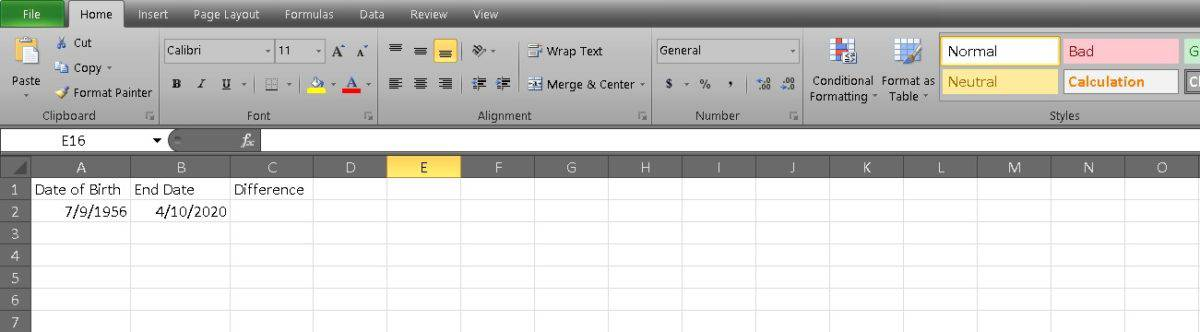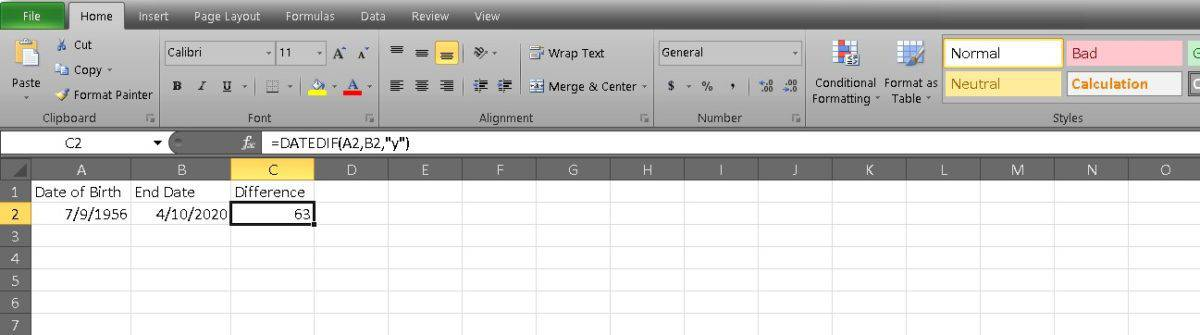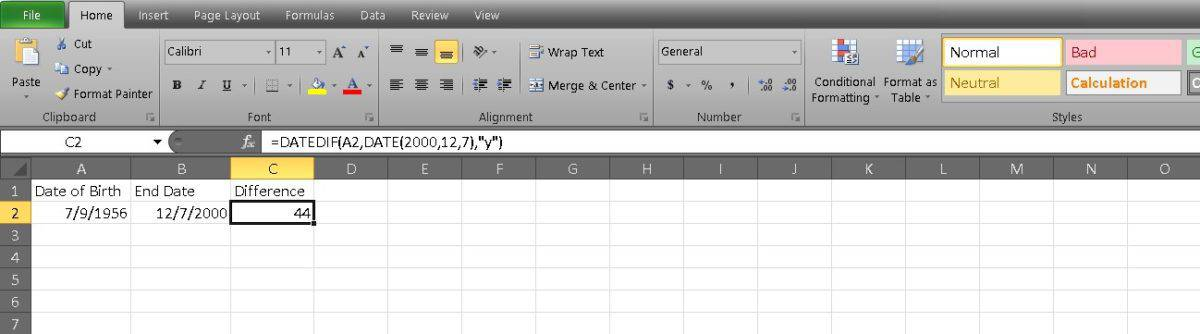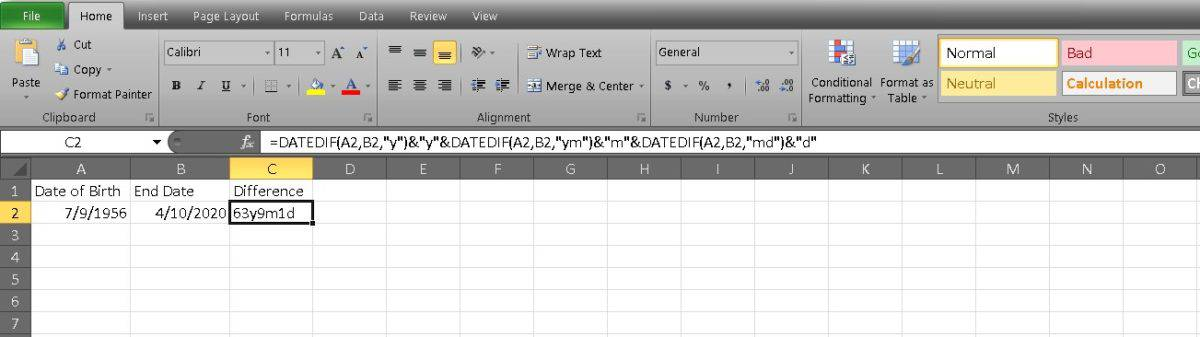Best daily deals

# How to calculate age in Excel

Curious how old someone is and too afraid to ask them? Here's a trick to find out.
By
February 16, 2023

Finding the exact difference between two dates in Excel may not be the most used feature, but it can be a lot of fun. A classic example is working out someone’s age. It’s not as complicated as it sounds, and there’s a handy function in Excel that can give you an exact age to the day. Read on for tips on how to calculate age in Excel.

To calculate someone's age in Excel, enter the formula =DATEDIF(A2,TODAY()," y"), where cell A2 contains the date they were born and "Today" is today's date. Press Enter, and Excel will output the age.

## How to calculate age in Excel

This is a pretty simple three-column task. To get started, enter the date of a person’s birth into your first cell. In our example, we’re using Tom Hanks, whose date of birth is in cell A2.

Now, enter today’s date into the cell next to your first cell. It’s just easier to keep both dates together if you’re tracking multiple ages.In the third cell (for us, it’s C2), enter the following formula:

Code
``=DATEDIF(A2, B2, "y").``

The two cell identifiers are relatively straightforward, and using the letter y as the third indicator means that you’re only interested in the number of years.You can also get a person’s age without entering today’s date in the second cell. To do this, change your formula to =DATEDIF(A2,TODAY(),” y”). Excel will do the rest by identifying the date and calculating the age.

If you really want to get specific, you can also calculate a person’s age on a particular historical date. We’re going to get historical with our example and calculate the exact age of Tom Hanks when the film Castaway was released: December 7, 2000.

The formula should look like this:

Code
``=DATEDIF(A2, DATE(2000,12,7), "y")``

Note that the date format is year, month, and day.The final, most specific measurement you can make is a person’s age, including months and days. The formula takes a little longer than previous measurements, but the process remains the same. Your formula should look like this:

Code
``=DATEDIF(A2,B2, “y”) & “y” & DATEDIF(A2, B2, “ym”) & “m” & DATEDIF(A2,B2, “md”) & “d.”``While it looks like a mouthful, it makes more sense once you break it down. You need to have a DATEDIF function for each level of measurement, which means that the first function indicates years. The second function measures the years and months, but the “m” ensures that it only displays the month. The final function calculates the differences in months and days without the years and shows the date with “d.”

Now you know how to calculate age in Excel. Please only use your powers for good.